# 看完就懂了！一篇搞定图论最短路径问题

## 最最原始的问题——两点间的最短路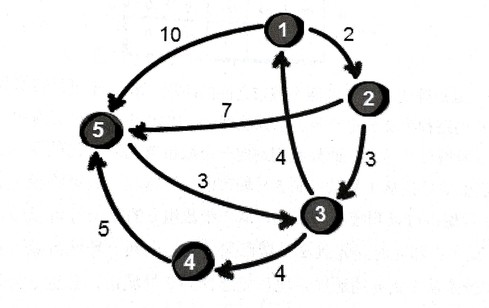void dfs(int cur, int dis) //cur-当前所在城市编号，dis-当前已走过的路径
{
if(dis > min) return; //若当前路径已比之前找到的最短路大，没必要继续尝试（一个小优化，可以不写）
if(cur == n) //当前已到达目的城市，更新min
{
if(dis < min) min = dis;
return;
}

for(int i = 1; i <= n; i++) //对1~n号城市依次尝试
{
if(e[cur][i] != INF && book[i] == 0) //若cur与i可达，且i没有在已走过的路径中
{
book[i] = 1; //标记i为已在路径中
dfs(i, dis+e[cur][i]); //继续搜索
book[i] = 0; //对从i出发的路径探索完毕，取消标记
}
}
}


void dfs(int step)
{
判断边界
尝试每一种可能 for(int i = 1; i <= n; i++)
{
继续下一步 dfs(step+1)
}
}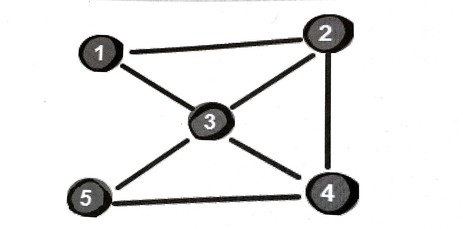int bfs()
{
queue<pair<int,int>> que; //pair记录城市编号和dis，也可以用结构体
que.push({1,0}); //把起始点加入队列
book = 1; //标记为已在路径中
while(!que.empty())
{
int cur = que.front();
que.pop();
for(int i = 1; i <= n; i++)
{
if(e[cur][i] != MAX && book[i] == 0) //若从cur到i可达且i不在队列中，i入队
{
que.push({i, cur.second+1});
book[i] = 1;
if(i == n) return cur.second; //如果已扩展出目标结点了，返回中转城市数答案
}
}
}
}


## 膨胀——任意两点间的最短路for(int i = 1; i <= n; i++)
for(int j = 1; j <= n; j++)
{
if(e[i][j] > e[i]+e[j]) e[i][j] = e[i]+e[j];
}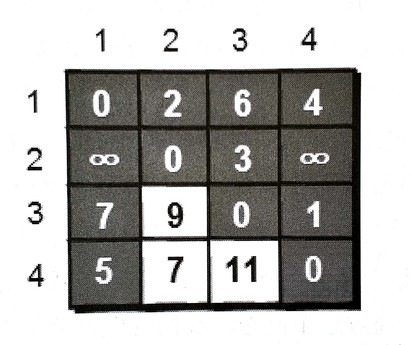for(int k = 1; k <= n; k++)
for(int i = 1; i <= n; i++)
for(int j = 1; j <= n; j++)
if(e[i][j] > e[i][k]+e[k][j])
e[i][j] = e[i][k]+e[k][j];


## 最常见的问题——单源最短路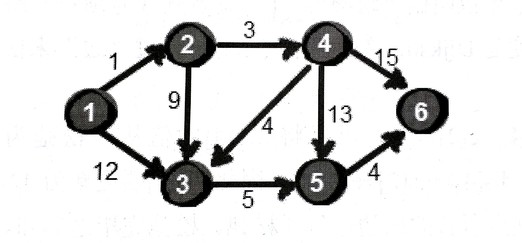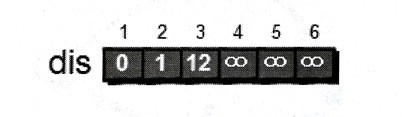balabala，这样一直做下去直到已经没有“剩下的”结点，算法结束。

1. 所有结点分为两部分：已确定最短路的结点集合P、未知最短路的结点集合Q。最开始，P中只有源点这一个结点。（可用一个book数组来维护是否在P中）
2. 在Q中选取一个离源点最近的结点u（dis[u]最小）加入集合P。然后考察u的所有出边，做松弛操作。
3. 重复第二步，直到集合Q为空。最终dis数组的值就是源点到所有顶点的最短路。

for(int i = 1; i <= n; i++) dis[i] = e[i]; //初始化dis为源点到各点的距离
for(int i = 1; i <= n; i++) book[i] = 0;
book = 1; //初始时P集合中只有源点

for(int i = 1; i <= n-1; i++) //做n-1遍就能把Q遍历空
{
int min = INF;
int u;
for(int j = 1; j <= n; j++) //寻找Q中最近的结点
{
if(book[j] == 0 && dis[j] < min)
{
min = dis[j];
u = j;
}
}
book[u] = 1; //加入到P集合
for(int v = 1; v <= n; v++) //对u的所有出边进行松弛
{
if(e[u][v] < INF)
{
if(dis[v] > dis[u] + e[u][v])
dis[v] = dis[u] + e[u][v];
}
}
}


Dijkstra是一种基于贪心策略的算法。每次新扩展一个路径最短的点，更新与它相邻的所有点。当所有边权为正时，由于不会存在一个路程更短的没扩展过的点，所以这个点的路程就确定下来了，这保证了算法的正确性。

（用三个数组存边，第i条边表示u[i]->v[i]，权值为w[i]）

for(int k = 1; k <= n-1; k++)
for(int i = 1; i <= m; i++)
if(dis[v[i]] > dis[u[i]] + w[i])
dis[v[i]] = dis[u[i]] + w[i];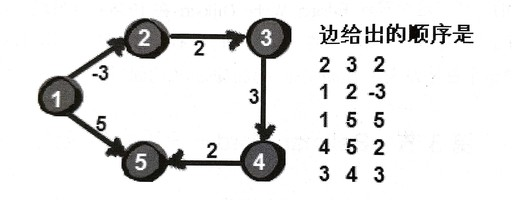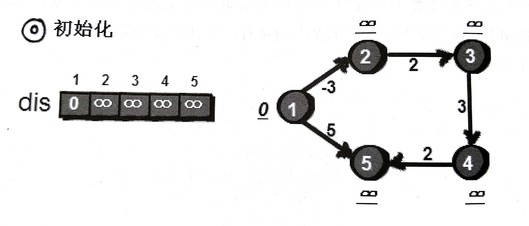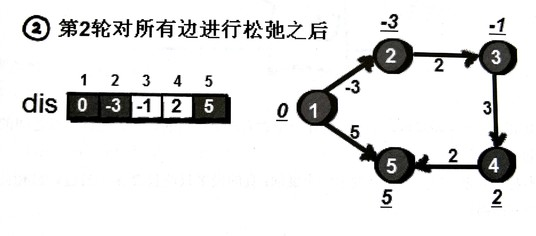for(int i = 1; i <= n; i++) dis[i] = INF;
dis = 0; //初始化dis数组，只有1号的距离为0

for(int k = 1; k <= n-1; k++) //进行n-1轮松弛
for(int i = 1; i <= m; i++) //枚举每一条边
if(dis[v[i]] > dis[u[i]] + w[i]) //尝试进行松弛
dis[v[i]] = dis[u[i]] + w[i];


for(int i = 1; i <= m; i++)
if(dis[v[i]] > dis[u[i]] + w[i]) flag = 1;


Bellman-Ford算法的时间复杂度是O(nm)，貌似比Dijkstra还高。事实上还可以进行优化，比如可以加一个bool变量check用来标记数组dis在本轮松弛中是否发生了变化，如果没有，就可以提前挑出循环。因为是“最多”达到n-1轮，实际情况下经常是早就已经达到最短，没法继续成功松弛了。

for(int k = 1; k <= n-1; k++) //进行n-1轮松弛
{
bool check = 0;
for(int i = 1; i <= m; i++) //枚举每一条边
if(dis[v[i]] > dis[u[i]] + w[i]) //尝试进行松弛
{
dis[v[i]] = dis[u[i]] + w[i];
check = 1;
}
if(check == 0) break;
}


1. 初始时将源点加入队列。
2. 每次选取队首结点u，对u的所有出边进行松弛。假设有一条边u->v松弛成功了，那就把v加入队列。然而，同一个结点同时在队列中出现多次是毫无意义的（可以用一个bool数组来判哪些结点在队列中）。所以刚提到的操作其实是，如果v不在当前队列中，才把它加入队列。
3. 对u的所有出边松弛完毕后，u出队。接下来不断的取出新的队首做第2步操作，直到队列为空。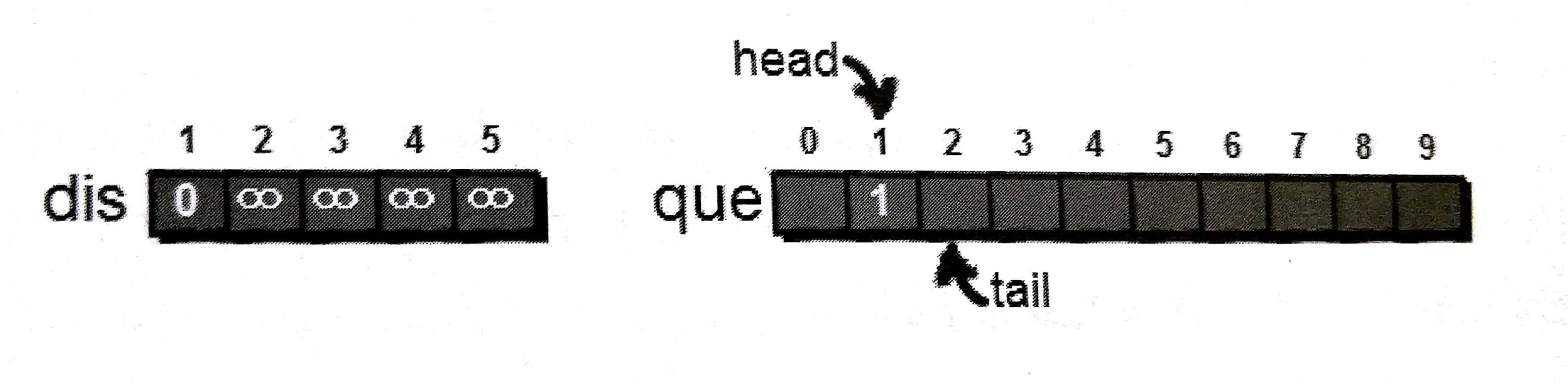1号结点处理完毕，此时将1号出队，接着对队首也就是2号结点进行同样的处理。在处理2->5这条边的时候，虽然松弛成功，dis从10更新为9了，但5号顶点已经在队列中，所以5号不能再次入队。for(int i = 1; i <= n; i++) book[i] = 0; //初始时都不在队列中
queue<int> que;
que.push(1); //将结点1加入队列
book = 1; //并打标记

while(!que.empty())
{
int cur = que.empty(); //取出队首
for(int i = 1; i <= n; i++)
{
if(e[cur][i] != INF && dis[i] > dis[cur]+e[cur][i]) //若cur到i有边且能够松弛
{
dis[i] = dis[cur]+e[cur][i]; //更新dis[i]
if(book[i] == 0) //若i不在队列中则加入队列
{
que.push(i);
book[i] = 1;
}
}
}

que.pop(); //队首出队
book[cur] = 0;
}


## 最短路径算法的对比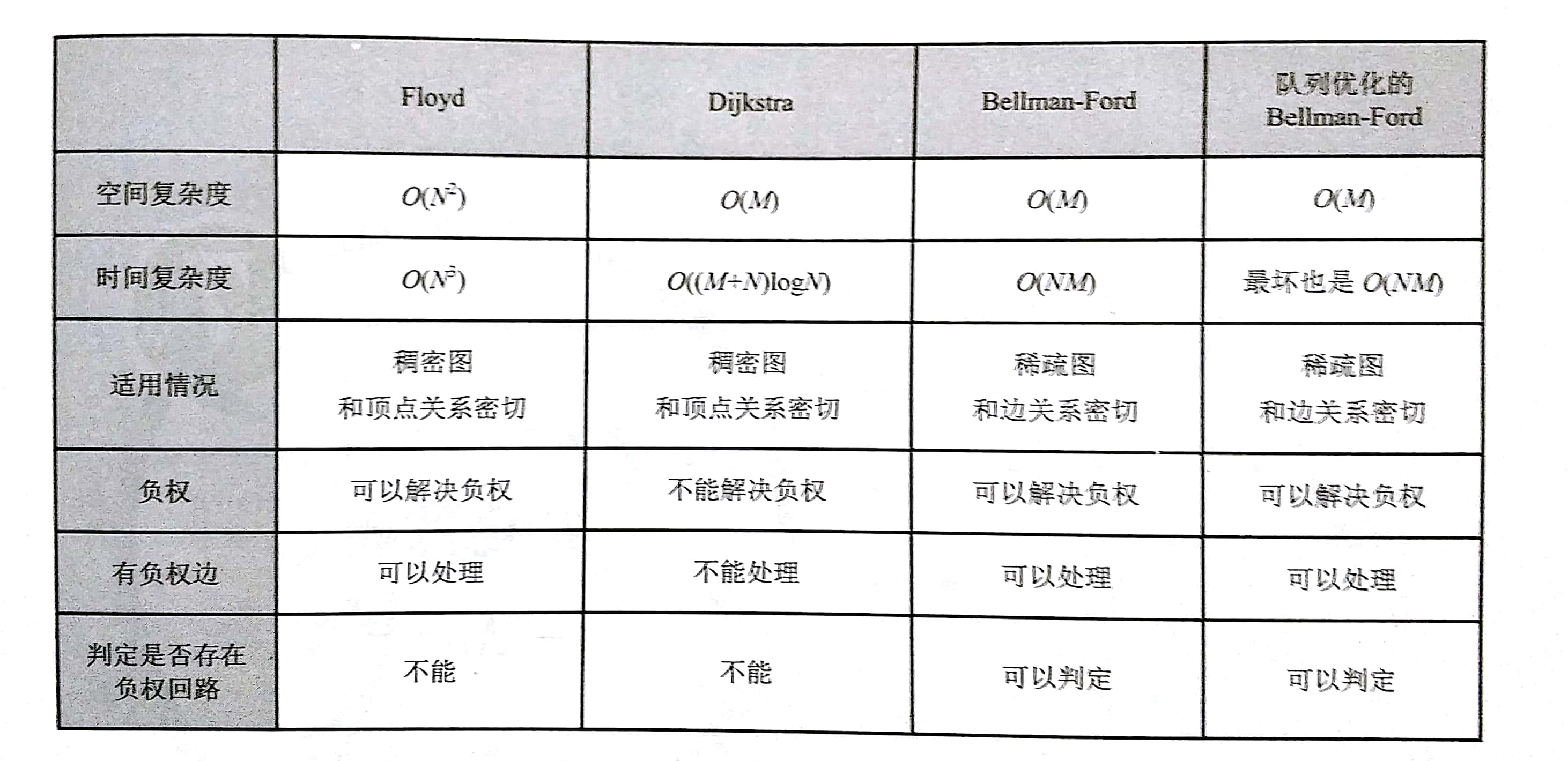## 附

posted @ 2018-06-26 15:34  thousfeet  阅读(37246)  评论(5编辑  收藏  举报
/* */